#Function Repository Resource:

# MultisetCardinality

Determine the cardinality for a multiset

Contributed by: Robert B. Nachbar (Wolfram Solutions)
 ResourceFunction["MultisetCardinality"][list] gives the cardinality of the multiset list. ResourceFunction["MultisetCardinality"][assoc] gives the cardinality of the Association multiset assoc, effectively giving the total of the values.

## Details and Options

The cardinality of a set is the number of elements it contains.
The list need not have the head List.
The values of assoc must be counts—that is non-negative Integer values.

## Examples

### Basic Examples

Give the cardinality:

 In:=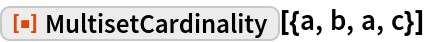Out=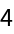In:=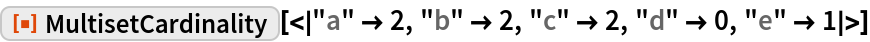Out=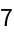### Scope

MultisetCardinality works with any head, not just List:

 In:=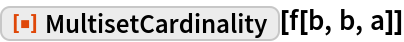Out=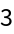### Properties and Relations

Compare to Length:

 In:=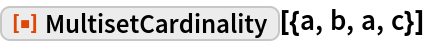Out=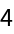In:=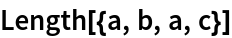Out=Compare to Total:

 In:=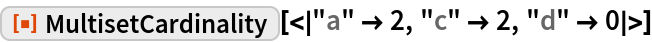Out=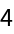In:=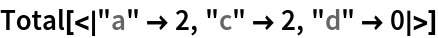Out=## Requirements

Wolfram Language 11.3 (March 2018) or above